Anzeige

# Optimal Sizing and Design of Isolated Micro-Grid systems

8. Feb 2023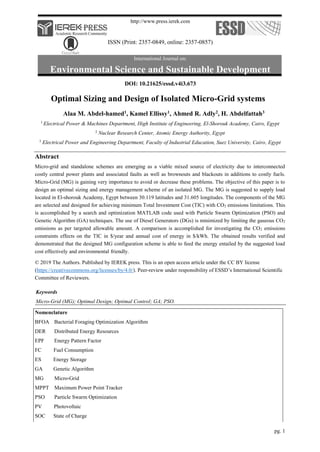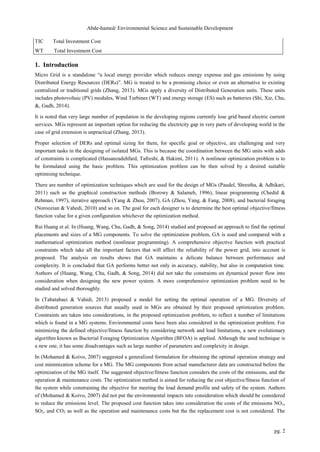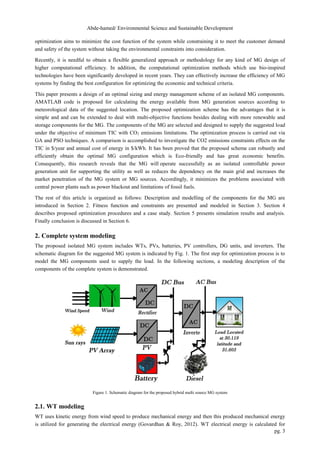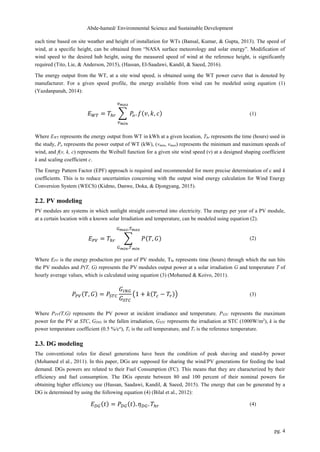Anzeige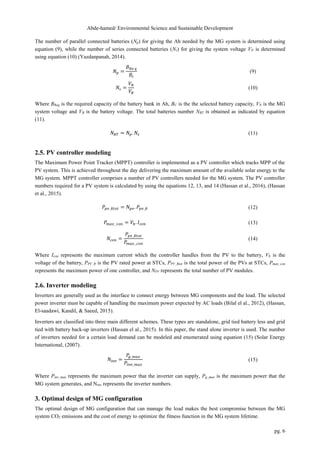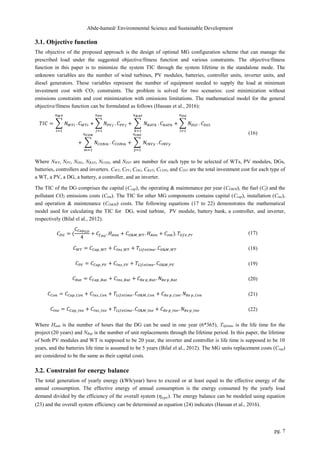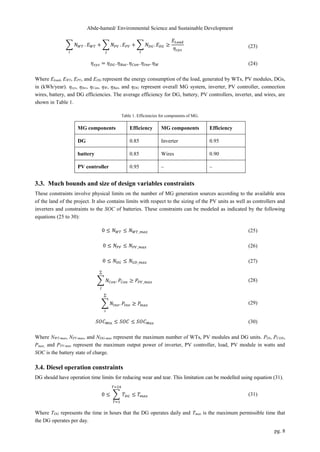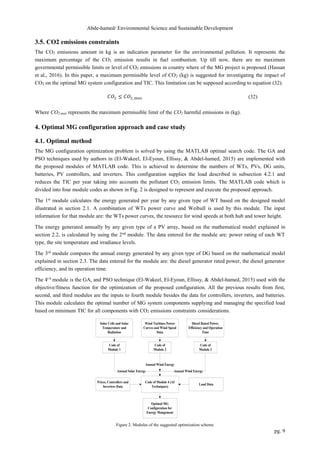Anzeige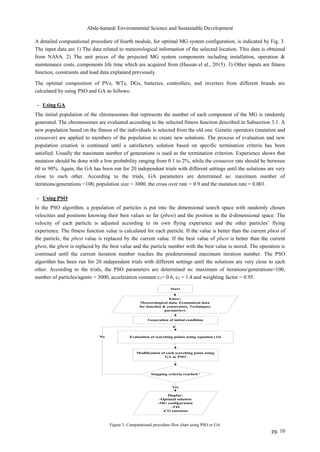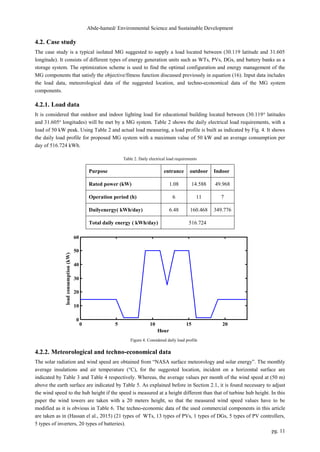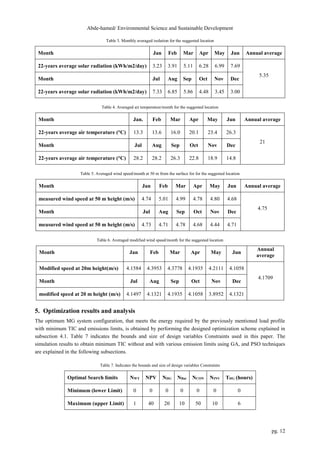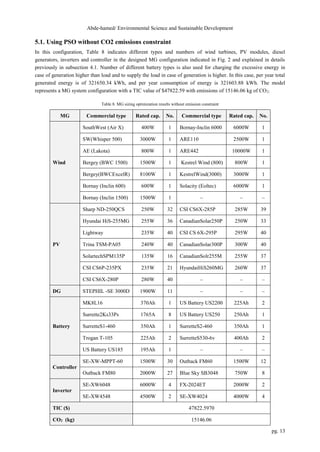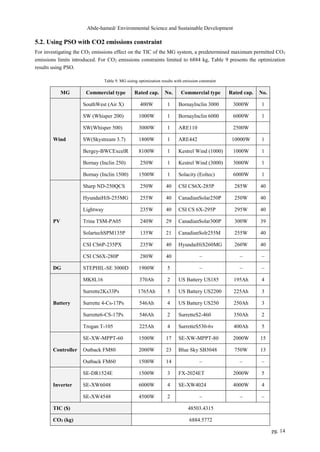Anzeige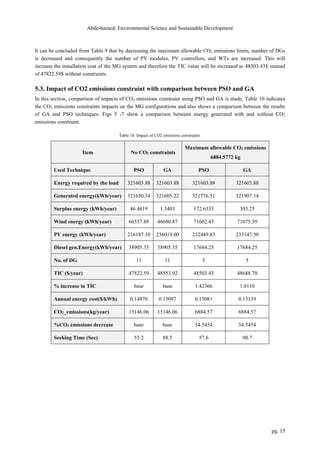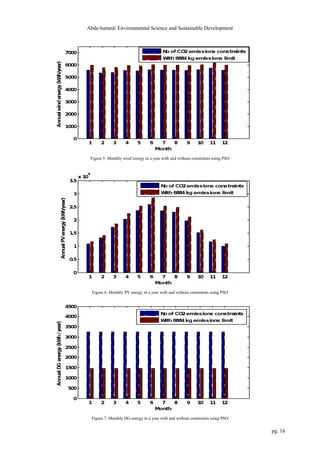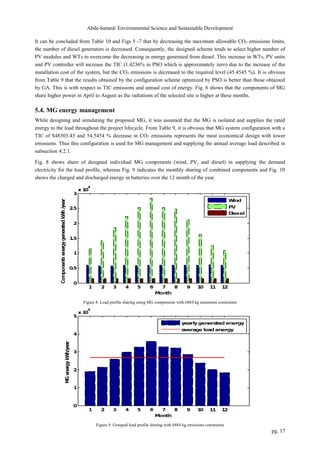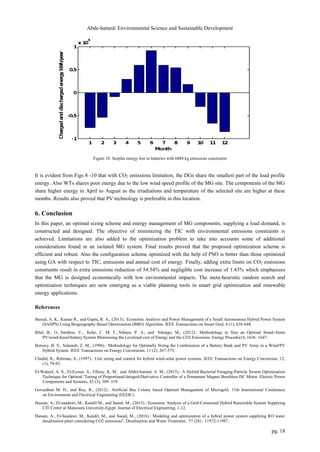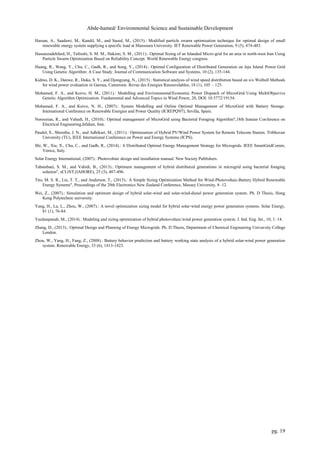Nächste SlideShare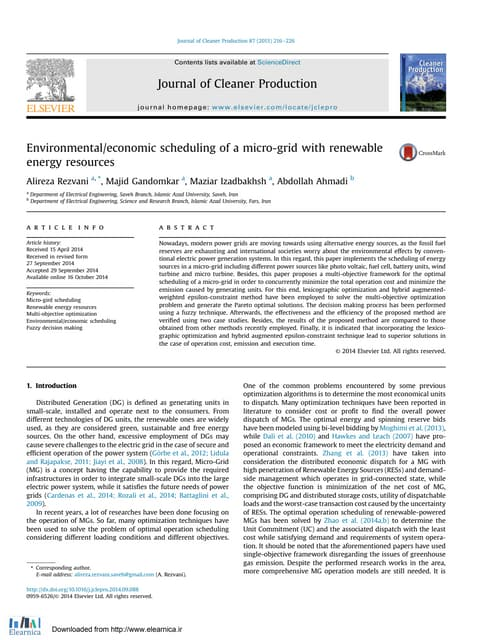JCP
1 von 19
Anzeige

### Optimal Sizing and Design of Isolated Micro-Grid systems

3. Abde-hamed/ Environmental Science and Sustainable Development pg. 3 optimization aims to minimize the cost function of the system while constraining it to meet the customer demand and safety of the system without taking the environmental constraints into consideration. Recently, it is needful to obtain a flexible generalized approach or methodology for any kind of MG design of higher computational efficiency. In addition, the computational optimization methods which use bio-inspired technologies have been significantly developed in recent years. They can effectively increase the efficiency of MG systems by finding the best configuration for optimizing the economic and technical criteria. This paper presents a design of an optimal sizing and energy management scheme of an isolated MG components. AMATLAB code is proposed for calculating the energy available from MG generation sources according to meteorological data of the suggested location. The proposed optimization scheme has the advantages that it is simple and and can be extended to deal with multi-objective functions besides dealing with more renewable and storage components for the MG. The components of the MG are selected and designed to supply the suggested load under the objective of minimum TIC with CO2 emissions limitations. The optimization process is carried out via GA and PSO techniques. A comparison is accomplished to investigate the CO2 emissions constraints effects on the TIC in \$/year and annual cost of energy in \$/kWh. It has been proved that the proposed scheme can robustly and efficiently obtain the optimal MG configuration which is Eco-friendly and has great economic benefits. Consequently, this research reveals that the MG will operate successfully as an isolated controllable power generation unit for supporting the utility as well as reduces the dependency on the main grid and increases the market penetration of the MG system or MG sources. Accordingly, it minimizes the problems associated with central power plants such as power blackout and limitations of fossil fuels. The rest of this article is organized as follows: Description and modelling of the components for the MG are introduced in Section 2. Fitness function and constraints are presented and modeled in Section 3. Section 4 describes proposed optimization procedures and a case study. Section 5 presents simulation results and analysis. Finally conclusion is discussed in Section 6. 2. Complete system modeling The proposed isolated MG system includes WTs, PVs, batteries, PV controllers, DG units, and inverters. The schematic diagram for the suggested MG system is indicated by Fig. 1. The first step for optimization process is to model the MG components used to supply the load. In the following sections, a modeling description of the components of the complete system is demonstrated. Figure 1. Schematic diagram for the proposed hybrid multi source MG system 2.1. WT modeling WT uses kinetic energy from wind speed to produce mechanical energy and then this produced mechanical energy is utilized for generating the electrical energy (Govardhan & Roy, 2012). WT electrical energy is calculated for
4. Abde-hamed/ Environmental Science and Sustainable Development pg. 4 each time based on site weather and height of installation for WTs (Bansal, Kumar, & Gupta, 2013). The speed of wind, at a specific height, can be obtained from “NASA surface meteorology and solar energy”. Modification of wind speed to the desired hub height, using the measured speed of wind at the reference height, is significantly required (Tito, Lie, & Anderson, 2015), (Hassan, El-Saadawi, Kandil, & Saeed, 2016). The energy output from the WT, at a site wind speed, is obtained using the WT power curve that is denoted by manufacturer. For a given speed profile, the energy available from wind can be modeled using equation (1) (Yazdanpanah, 2014): 𝐸𝑊𝑇 = 𝑇ℎ𝑟 ∑ 𝑃 𝑜. 𝑓(𝑣, 𝑘, 𝑐) 𝑣𝑚𝑎𝑥 𝑣𝑚𝑖𝑛 (1) Where EWT represents the energy output from WT in kWh at a given location, Thr represents the time (hours) used in the study, Po represents the power output of WT (kW), (vmin, vmax) represents the minimum and maximum speeds of wind, and f(v, k, c) represents the Weibull function for a given site wind speed (ν) at a designed shaping coefficient k and scaling coefficient c. The Energy Pattern Factor (EPF) approach is required and recommended for more precise determination of c and k coefficients. This is to reduce uncertainties concerning with the output wind energy calculation for Wind Energy Conversion System (WECS) (Kidmo, Danwe, Doka, & Djongyang, 2015). 2.2. PV modeling PV modules are systems in which sunlight straight converted into electricity. The energy per year of a PV module, at a certain location with a known solar Irradiation and temperature, can be modeled using equation (2). 𝐸𝑃𝑉 = 𝑇ℎ𝑟 ∑ 𝑃(𝑇, 𝐺) 𝐺𝑚𝑎𝑥,𝑇𝑚𝑎𝑥 𝐺𝑚𝑖𝑛,𝑇𝑚𝑖𝑛 (2) Where EPV is the energy production per year of PV module, Thr represents time (hours) through which the sun hits the PV modules and P(T, G) represents the PV modules output power at a solar irradiation G and temperature T of hourly average values, which is calculated using equation (3) (Mohamed & Koivo, 2011). 𝑃𝑃𝑉(𝑇, 𝐺) = 𝑃𝑆𝑇𝐶 𝐺𝐼𝑁𝐺 𝐺𝑆𝑇𝐶 (1 + 𝑘(𝑇𝑐 − 𝑇𝑟)) (3) Where PPV(T,G) represents the PV power at incident irradiance and temperature, PSTC represents the maximum power for the PV at STC, GING is the fallen irradiation, GSTC represents the irradiation at STC (1000W/m2 ), k is the power temperature coefficient (0.5 %/co ), Tc is the cell temperature, and Tr is the reference temperature. 2.3. DG modeling The conventional roles for diesel generations have been the condition of peak shaving and stand-by power (Mohamed el al., 2011). In this paper, DGs are supposed for sharing the wind/PV generations for feeding the load demand. DGs powers are related to their Fuel Consumption (FC). This means that they are characterized by their efficiency and fuel consumption. The DGs operate between 80 and 100 percent of their nominal powers for obtaining higher efficiency use (Hassan, Saadawi, Kandil, & Saeed, 2015). The energy that can be generated by a DG is determined by using the following equation (4) (Bilal et al., 2012): 𝐸𝐷𝐺(𝑡) = 𝑃𝐷𝐺(𝑡). 𝜂𝐷𝐺. 𝑇ℎ𝑟 (4)
5. Abde-hamed/ Environmental Science and Sustainable Development pg. 5 Where EDG is the DG energy per year in KWh, PDG is the DG rating power, 𝜂𝐷𝐺 is the DG efficiency, and Thr represents its hours of operations. The fuel consumption of a DG depends on both the load and the size of generator. Hourly fuel consumption is given by equation (5) (Hassan et al., 2016). 𝐹𝐶(𝑡) = 𝑎. 𝑃𝑜(𝑡) + 𝑏. 𝑃 𝑛 (5) Where, (a & b) represents the coefficients for the fuel consumption curve and ( Po & Pn) are power output and nominal rating of the DG. In this paper, a is taken to be 0.081451 L/kWh while b is taken to be 0.2461 L/kWh (Hassan et al., 2016). The total CO2 emission amount can be determined using the following equation (6) (Hassan et al., 2016): 𝑄𝑐𝑜2 = 𝐹𝐶 . 𝐸𝐹 (6) Where QCO2 is the total CO2 emission amount in (kg), FC is the fuel consumption in (kWh) and EF represents the emission factor for the fuel used in kg/kWh. For the diesel fuel considered in this article, the default CO2 emission factor is 0.705 kg/kWh (Hassan et al., 2016). Usage of a DG is not in line with prevention of air-pollution and minimization of CO2 emission. A gas micro- turbine will be more environmentally friendly solution but it is not practical to be used in our location and its cost is higher besides its complex design. A tax on CO2 levels of emissions in any sector did not yet applied by whether industrial or energy production in the site of the proposed MG. However, the department of environment stated, depending on the Environmental Law4 of 1994, that there is a need to force such a tax for emissions per year of that pollutant and harmful gas. The department of environment enumerated these ratings according to European standards. 2.4. Battery modeling Battery is defined as an electro-chemical device that stores the electrical energy from AC or DC units of MG for later use. Since the output of the renewable sources of the MG is a random behavior, the state of charge (SOC) of the battery is constantly changing accordingly in MG system. When the total power output from the WTS, PV modules is greater than the load power, the battery is in the SOC. When the total output power of the WT and PV modules is less than the load power, the battery is in the discharging state. The SOC of battery bank can be calculated from the following equation (7) (Wei, 2007): 𝑆𝑂𝐶(𝑡) = 𝑆𝑂𝐶(𝑡 − 1). (1 − 𝜎) + [𝐸𝐺𝑡(𝑡) − 𝐸𝐿(𝑡) 𝜂𝑖𝑛𝑣 ] . 𝜂𝑏𝑎𝑡 (7) Where, SOC(t) and SOC(t-1) are the battery bank state of charge at time t and t-1, σ is monthly self-discharging rate, EGt(t) is the total energy generated, EL(t) is the load demand, ηinv and ηbat are the efficiency of inverter and battery. In this paper, the battery SOC model is designed based on the Ah method. The capacity of battery bank (BReq) required for a MG system can be calculated using the following equation (8) (Hassan et al., 2016). 𝐵𝑅𝑒 𝑞 = 𝐿𝐴ℎ/𝑑𝑎𝑦. 𝑁𝑐 𝑀𝐷𝐷. 𝐷𝑓 (8) Where LAh/d is the total Ah consumption of the load per day, MDD is the maximum discharge depth and DF is the factor of discharging and NC represents the autonomous day’s number.
6. Abde-hamed/ Environmental Science and Sustainable Development pg. 6 The number of parallel connected batteries (Np) for giving the Ah needed by the MG system is determined using equation (9), while the number of series connected batteries (Ns) for giving the system voltage VN is determined using equation (10) (Yazdanpanah, 2014). 𝑁𝑝 = 𝐵𝑅𝑒 𝑞 𝐵𝑐 (9) 𝑁𝑠 = 𝑉𝑁 𝑉𝐵 (10) Where BReq is the required capacity of the battery bank in Ah, BC is the the selected battery capacity, VN is the MG system voltage and VB is the battery voltage. The total batteries number NBT is obtained as indicated by equation (11). 𝑁𝐵𝑇 = 𝑁𝑝. 𝑁𝑠 (11) 2.5. PV controller modeling The Maximum Power Point Tracker (MPPT) controller is implemented as a PV controller which tracks MPP of the PV system. This is achieved throughout the day delivering the maximum amount of the available solar energy to the MG system. MPPT controller comprises a number of PV controllers needed for the MG system. The PV controller numbers required for a PV system is calculated by using the equations 12, 13, and 14 (Hassan et al., 2016), (Hassan et al., 2015). 𝑃𝑝𝑣_𝑅𝑡𝑜𝑡 = 𝑁𝑝𝑣. 𝑃𝑝𝑣_𝑅 (12) 𝑃𝑚𝑎𝑥_ 𝑐𝑜𝑛 = 𝑉𝑏. 𝐼𝑐𝑜𝑛 (13) 𝑁𝑐𝑜𝑛 = 𝑃𝑝𝑣_𝑅𝑡𝑜𝑡 𝑃𝑚𝑎𝑥_ 𝑐𝑜𝑛 (14) Where Icon represents the maximum current which the controller handles from the PV to the battery, Vb is the voltage of the battery, PPV_R is the PV rated power at STCs, PPV_Rtot is the total power of the PVs at STCs, Pmax_con represents the maximum power of one controller, and NPV represents the total number of PV modules. 2.6. Inverter modeling Inverters are generally used as the interface to connect energy between MG components and the load. The selected power inverter must be capable of handling the maximum power expected by AC loads (Bilal el al., 2012), (Hassan, El-saadawi, Kandil, & Saeed, 2015). Inverters are classified into three main different schemes. These types are standalone, grid tied battery less and grid tied with battery back-up inverters (Hassan el al., 2015). In this paper, the stand alone inverter is used. The number of inverters needed for a certain load demand can be modeled and enumerated using equation (15) (Solar Energy International, (2007). 𝑁𝑖𝑛𝑣 = 𝑃 𝑔_𝑚𝑎𝑥 𝑃𝑖𝑛𝑣_𝑚𝑎𝑥 (15) Where Pinv_max represents the maximum power that the inverter can supply, Pg_max is the maximum power that the MG system generates, and Ninv represents the inverter numbers. 3. Optimal design of MG configuration The optimal design of MG configuration that can manage the load makes the best compromise between the MG system CO2 emissions and the cost of energy to optimize the fitness function in the MG system lifetime.
7. Abde-hamed/ Environmental Science and Sustainable Development pg. 7 3.1. Objective function The objective of the proposed approach is the design of optimal MG configuration scheme that can manage the prescribed load under the suggested objective/fitness function and various constraints. The objective/fitness function in this paper is to minimize the system TIC through the system lifetime in the standalone mode. The unknown variables are the number of wind turbines, PV modules, batteries, controller units, inverter units, and diesel generators. These variables represent the number of equipment needed to supply the load at minimum investment cost with CO2 constraints. The problem is solved for two scenarios: cost minimization without emissions constraints and cost minimization with emissions limitations. The mathematical model for the general objective/fitness function can be formulated as follows (Hassan et al., 2016): 𝑇𝐼𝐶 = ∑ 𝑁𝑊𝑇𝑖 𝑛𝑊𝑇 𝑖=1 . 𝐶𝑊𝑇𝑖 + ∑ 𝑁𝑃𝑉𝑗 𝑛𝑃𝑉 𝑗=1 . 𝐶𝑃𝑉𝑗 + ∑ 𝑁𝐵𝐴𝑇𝑘 𝑛𝐵𝐴𝑇 𝑘=1 . 𝐶𝐵𝐴𝑇𝑘 + ∑ 𝑁𝐷𝐺𝑙 𝑛𝐷𝐺 𝑙=1 . 𝐶𝐷𝐺𝑙 + ∑ 𝑁𝐶𝑂𝑁𝑚 𝑛𝐶𝑂𝑁 𝑚=1 . 𝐶𝐶𝑂𝑁𝑚 + ∑ 𝑁𝐼𝑁𝑉𝑦 𝑛𝐼𝑁𝑉 𝑦=1 . 𝐶𝐼𝑁𝑉𝑦 (16) Where NWT, NPV, NDG, NBAT, NCON, and NINV are number for each type to be selected of WTs, PV modules, DGs, batteries, controllers and inverters. CWT, CPV, CDG, CBAT, CCON, and CINV are the total investment cost for each type of a WT, a PV, a DG, a battery, a controller, and an inverter. The TIC of the DG comprises the capital (Ccap), the operating & maintenance per year (CO&M), the fuel (Cf) and the pollutant CO2 emissions costs (Cem). The TIC for other MG components contains capital (Ccap), installation (Cins), and operation & maintenance (CO&M) costs. The following equations (17 to 22) demonstrates the mathematical model used for calculating the TIC for DG, wind turbine, PV module, battery bank, a controller, and inverter, respectively (Bilal el al., 2012). 𝐶𝐷𝐺 = ( 𝐶𝐶𝑎𝑝𝐺𝐷 4 + 𝐶𝑓𝐷𝐺 . 𝐻𝐴𝑛𝑛 + 𝐶𝑂&𝑀_𝑊𝑇. 𝐻𝐴𝑛𝑛 + 𝐶𝑒𝑚). 𝑇𝑙𝑖𝑓𝑒_𝑃𝑟 (17) 𝐶𝑊𝑇 = 𝐶𝐶𝑎𝑝_𝑊𝑇 + 𝐶𝐼𝑛𝑠_𝑊𝑇 + 𝑇𝐿𝑖𝑓𝑒𝑡𝑖𝑚𝑒. 𝐶𝑂&𝑀_𝑊𝑇 (18) 𝐶𝑃𝑉 = 𝐶𝐶𝑎𝑝_𝑃𝑉 + 𝐶𝐼𝑛𝑠_𝑃𝑉 + 𝑇𝐿𝑖𝑓𝑒𝑡𝑖𝑚𝑒. 𝐶𝑂&𝑀_𝑃𝑉 (19) 𝐶𝐵𝑎𝑡 = 𝐶𝐶𝑎𝑝_𝐵𝑎𝑡 + 𝐶𝐼𝑛𝑠_𝐵𝑎𝑡 + 𝐶𝑅𝑒 𝑝_𝐵𝑎𝑡. 𝑁𝑅𝑒 𝑝_𝐵𝑎𝑡 (20) 𝐶𝐶𝑜𝑛 = 𝐶𝐶𝑎𝑝_𝐶𝑜𝑛 + 𝐶𝐼𝑛𝑠_𝐶𝑜𝑛 + 𝑇𝐿𝑖𝑓𝑒𝑡𝑖𝑚𝑒. 𝐶𝑂&𝑀_𝐶𝑜𝑛 + 𝐶𝑅𝑒 𝑝_𝐶𝑜𝑛. 𝑁𝑅𝑒 𝑝_𝐶𝑜𝑛 (21) 𝐶𝐼𝑛𝑣 = 𝐶𝐶𝑎𝑝_𝐼𝑛𝑣 + 𝐶𝐼𝑛𝑠_𝐼𝑛𝑣 + 𝑇𝐿𝑖𝑓𝑒𝑡𝑖𝑚𝑒. 𝐶𝑂&𝑀_𝐼𝑛𝑣 + 𝐶𝑅𝑒 𝑝_𝐼𝑛𝑣. 𝑁𝑅𝑒 𝑝_𝐼𝑛𝑣 (22) Where Hann is the number of hours that the DG can be used in one year (6*365), Tlifetime is the life time for the project (20 years) and NRep is the number of unit replacements through the lifetime period. In this paper, the lifetime of both PV modules and WT is supposed to be 20 year, the inverter and controller is life time is supposed to be 10 years, and the batteries life time is assumed to be 5 years (Bilal el al., 2012). The MG units replacement costs (Crep) are considered to be the same as their capital costs. 3.2. Constraint for energy balance The total generation of yearly energy (kWh/year) have to exceed or at least equal to the effective energy of the annual consumption. The effective energy of annual consumption is the energy consumed by the yearly load demand divided by the efficiency of the overall system (𝜂𝑠𝑦𝑠). The energy balance can be modeled using equation (23) and the overall system efficiency can be determined as equation (24) indicates (Hassan et al., 2016).
8. Abde-hamed/ Environmental Science and Sustainable Development pg. 8 ∑ 𝑁𝑊𝑇 𝑖 . 𝐸𝑊𝑇 + ∑ 𝑁𝑃𝑉 𝑗 . 𝐸𝑃𝑉 + ∑ 𝑁𝐷𝐺. 𝐸𝐷𝐺 ≥ 𝐸𝐿𝑜𝑎𝑑 𝜂𝑠𝑦𝑠 𝑙 (23) 𝜂𝑠𝑦𝑠 = 𝜂𝐷𝐺. 𝜂𝐵𝑎𝑡. 𝜂𝐶𝑜𝑛. 𝜂𝐼𝑛𝑣. 𝜂𝑊 (24) Where Eload, EWT, EPV, and EDG represent the energy consumption of the load, generated by WTs, PV modules, DGs, in (kWh/year). ηsys, ηInv, ηCon, ηW, ηBat, and ηDG represent overall MG system, inverter, PV controller, connection wires, battery, and DG efficiencies. The average efficiency for DG, battery, PV controllers, inverter, and wires, are shown in Table 1. Table 1. Efficiencies for components of MG. MG components Efficiency MG components Efficiency DG 0.85 Inverter 0.95 battery 0.85 Wires 0.90 PV controller 0.95 – – 3.3. Much bounds and size of design variables constraints These constraints involve physical limits on the number of MG generation sources according to the available area of the land of the project. It also contains limits with respect to the sizing of the PV units as well as controllers and inverters and constraints to the SOC of batteries. These constraints can be modeled as indicated by the following equations (25 to 30): 0 ≤ 𝑁𝑊𝑇 ≤ 𝑁𝑊𝑇_𝑚𝑎𝑥 (25) 0 ≤ 𝑁𝑃𝑉 ≤ 𝑁𝑃𝑉_𝑚𝑎𝑥 (26) 0 ≤ 𝑁𝐷𝐺 ≤ 𝑁𝐺𝐷_𝑚𝑎𝑥 (27) ∑ 𝑁𝐶𝑜𝑛. 𝑃𝐶𝑜𝑛 ≥ 𝑃𝑃𝑉_𝑚𝑎𝑥 ∑ 𝑗 (28) ∑ 𝑁𝐼𝑛𝑣. 𝑃𝐼𝑛𝑣 ≥ 𝑃𝑚𝑎𝑥 ∑ 𝑖 (29) 𝑆𝑂𝐶𝑀𝑖𝑛 ≤ 𝑆𝑂𝐶 ≤ 𝑆𝑂𝐶𝑀𝑎𝑥 (30) Where NWT-max, NPV-max, and NDG-max represent the maximum number of WTs, PV modules and DG units. PIN, PCON, Pmax, and PPV-max represent the maximum output power of inverter, PV controller, load, PV module in watts and SOC is the battery state of charge. 3.4. Diesel operation constraints DG should have operation time limits for reducing wear and tear. This limitation can be modelled using equation (31). 0 ≤ ∑ 𝑇𝐷𝐺 𝑇=24 𝑇=1 ≤ 𝑇𝑚𝑎𝑥 (31) Where TDG represents the time in hours that the DG operates daily and Tmax is the maximum permissible time that the DG operates per day.
9. Abde-hamed/ Environmental Science and Sustainable Development pg. 9 3.5. CO2 emissions constraints The CO2 emissions amount in kg is an indication parameter for the environmental pollution. It represents the maximum percentage of the CO2 emission results in fuel combustion. Up till now, there are no maximum governmental permissible limits or level of CO2 emissions in country where of the MG project is proposed (Hassan et al., 2016). In this paper, a maximum permissible level of CO2 (kg) is suggested for investigating the impact of CO2 on the optimal MG system configuration and TIC. This limitation can be supposed according to equation (32). 𝐶𝑂2 ≤ 𝐶𝑂2_𝑚𝑎𝑥 (32) Where CO2-max represents the maximum permissible limit of the CO2 harmful emissions in (kg). 4. Optimal MG configuration approach and case study 4.1. Optimal method The MG configuration optimization problem is solved by using the MATLAB optimal search code. The GA and PSO techniques used by authors in (El-Wakeel, El-Eyoun, Ellissy, & Abdel-hamed, 2015) are implemented with the proposed modules of MATLAB code. This is achieved to determine the numbers of WTs, PVs, DG units, batteries, PV controllers, and inverters. This configuration supplies the load described in subsection 4.2.1 and reduces the TIC per year taking into accounts the pollutant CO2 emission limits. The MATLAB code which is divided into four module codes as shown in Fig. 2 is designed to represent and execute the proposed approach. The 1st module calculates the energy generated per year by any given type of WT based on the designed model illustrated in section 2.1. A combination of WTs power curve and Weibull is used by this module. The input information for that module are: the WTs power curves, the resource for wind speeds at both hub and tower height. The energy generated annually by any given type of a PV array, based on the mathematical model explained in section 2.2, is calculated by using the 2nd module. The data entered for the module are: power rating of each WT type, the site temperature and irradiance levels. The 3rd module computes the annual energy generated by any given type of DG based on the mathematical model explained in section 2.3. The data entered for the module are: the diesel generator rated power, the diesel generator efficiency, and its operation time. The 4t h module is the GA, and PSO technique (El-Wakeel, El-Eyoun, Ellissy, & Abdel-hamed, 2015) used with the objective/fitness function for the optimization of the proposed configuration. All the previous results from first, second, and third modules are the inputs to fourth module besides the data for controllers, inverters, and batteries. This module calculates the optimal number of MG system components supplying and managing the specified load based on minimum TIC for all components with CO2 emissions constraints considerations. Solar Cells and Solar Temperature and Radiation Code of Module 1 Code of Module 4 (AI Techniques) Prices, Controllers and Inverters Data Wind Turbines Power Curves and Wind Speed Data Diesel Rated Power, Efficiency and Operation Time Code of Module 2 Code of Module 3 Annual Solar Energy Annual Wind Energy Annual Wind Energy Load Data Optimal MG Configuration for Energy Mangement Figure 2. Modules of the suggested optimization scheme
10. Abde-hamed/ Environmental Science and Sustainable Development pg. 10 A detailed computational procedure of fourth module, for optimal MG system configuration, is indicated by Fig. 3. The input data are 1) The data related to meteorological information of the selected location. This data is obtained from NASA. 2) The unit prices of the projected MG system components including installation, operation & maintenance costs, components life time which are acquired from (Hassan el al., 2015). 3) Other inputs are fitness function, constraints and load data explained previously. The optimal composition of PVs, WTs, DGs, batteries, controllers, and inverters from different brands are calculated by using PSO and GA as follows: - Using GA The initial population of the chromosomes that represents the number of each component of the MG is randomly generated. The chromosomes are evaluated according to the selected fitness function described in Subsection 3.1. A new population based on the fitness of the individuals is selected from the old one. Genetic operators (mutation and crossover) are applied to members of the population to create new solutions. The process of evaluation and new population creation is continued until a satisfactory solution based on specific termination criteria has been satisfied. Usually the maximum number of generations is used as the termination criterion. Experience shows that mutation should be done with a low probability ranging from 0.1 to 2%, while the crossover rate should be between 60 to 90%. Again, the GA has been run for 20 independent trials with different settings until the solutions are very close to each other. According to the trials, GA parameters are determined as: maximum number of iterations/generations =100, population size = 3000, the cross over rate = 0.9 and the mutation rate = 0.001. - Using PSO In the PSO algorithm, a population of particles is put into the dimensional search space with randomly chosen velocities and positions knowing their best values so far (pbest) and the position in the d-dimensional space. The velocity of each particle is adjusted according to its own flying experience and the other particles’ flying experience. The fitness function value is calculated for each particle. If the value is better than the current pbest of the particle, the pbest value is replaced by the current value. If the best value of pbest is better than the current gbest, the gbest is replaced by the best value and the particle number with the best value is stored. The operation is continued until the current iteration number reaches the predetermined maximum iteration number. The PSO algorithm has been run for 20 independent trials with different settings until the solutions are very close to each other. According to the trials, the PSO parameters are determined as: maximum of iterations/generations=100, number of particles/agents = 3000, acceleration constant c1= 0.6, c2 = 1.4 and weighting factor = 0.95. Generation of initial condition Enter: Meteorological data, Economical data for function & constraints, Techniques parameters Evaluation of searching points using equation (14) Stopping criteria reached ˀ Modification of each searching point using GA or PSO Display: -Optimal solution -MG configuration -TIS -CO emssions Start Yes No Figure 3. Computational procedure flow chart using PSO or GA